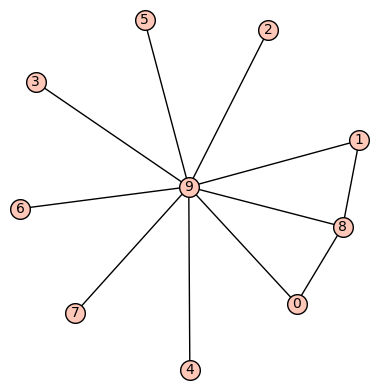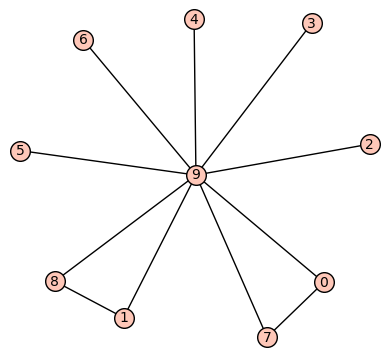# How to obtain the graph having highest algebraic connectivity?for G in graphs.nauty_geng("10-c"):

if G.size()==11:

L = G.laplacian_matrix().eigenvalues()

L.sort()

show(L)

G.show()

Using this code I have obtained the connected graphs on 10 vertices with 11 edges. Also I have obtained the Laplacian eigenvalues also for the corresponding graphs. The second smallest eigenvalue of Laplacian matrix is called the algebraic connectivity. Now among all these graphs, I need those graphs which have highest algebraic connectivity among all the graphs on 10 vertices with 11 edges. How we can do that?

edit retag close merge delete

Sort by » oldest newest most votedIt is just like last year:

algebraic_connectivity = lambda g: sorted(g.laplacian_matrix().eigenvalues())
G = max(graphs.nauty_geng("10 11:11 -c"), key=algebraic_connectivity)
GHere I also used nauty to restrict the number of edges to 11, for efficiency.

Of course there could be more than one such graph. Indeed, using the code from the old answer, the output is:

There are 2 graphs of (maximal) algebraic connectivity 1more

If possible, would you please give the whole code here so that I can obtain the two graphs. Thank you.

@Kuldeep You're welcome. In the old code, just put my_graphs = graphs.nauty_geng("10 11:11 -c") at the top, and replace print ... by print(...) (we need parentheses in now, in Python 3).

1

Now, if we run the same coder for 8 vertices and 9 edges, we should get three graphs having algebraic connectivity=1. But I am getting only 2. Where is the fault. will you please help?